Related Articles
Graph Plotting in R Programming
• Last Updated : 17 May, 2020

When it comes to interpreting the world and the enormous amount of data it is producing on a daily basis, Data Visualization becomes the most desirable way. Rather than screening huge Excel sheets, it is always better to visualize that data through charts and graphs, to gain meaningful insights.

The R Programming language provides some easy and quick tools that let us convert our data into visually insightful elements like graphs.

Graph plotting in R is of two types:

• One-dimensional Plotting: In one-dimensional plotting, we plot one variable at a time. For example, we may plot a variable with the number of times each of its values occurred in the entire dataset (frequency). So, it is not compared to any other variable of the dataset. These are the 4 major types of graphs that are used for One-dimensional analysis –
• Five Point Summary
• Box Plotting
• Histograms
• Bar Plotting
• Two-dimensional Plotting: In two-dimensional plotting, we visualize and compare one variable with respect to the other. For example, in a dataset of Air Quality measures, we would like to compare how the AQI varies with the temperature at a particular place. So, temperature and AQI are two different variables and we wish to see how one changes with respect to the other. These are the 3 major kinds of graphs used for such kinds of analysis –
• Box Plotting
• Histograms
• Scatter plots
• For the purpose of this article, we will use the default dataset (mtcars) that is provided by RStudio.

Open RStudio (or R Terminal) and start by loading the dataset. Type these commands in the console. This is a way to load the default datasets provided by R. (Any other dataset may also be downloaded and used)

```> library(datasets)
> data(mtcars)
```

To check if the data is correctly loaded, we run the following command on console:

```> head(mtcars)
```

Output: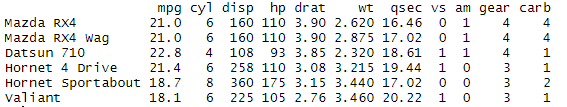By running this command, we also get to know what columns does our dataset contain. In this case, the dataset mtcars contains 11 columns namely – mpg, cyl, disp, hp, drat, wt, qsec, vs, am, gear, and carb. Note that the number of rows is larger than displayed here. `head()` function displays only the top 6 rows of the dataset.

### One-Dimensional Plotting

In one-dimensional plotting, we essentially plot one variable at a time. So, it is not compared to any other variable of the dataset. Rather, only its features of statistical inference are taken care of.

#### Five Point Summary

To reference a particular column name in R, we use the ‘\$’ sign. For example, if we want to refer to the ‘gear’ column in the mtcars dataset, we refer to it as – mtcars\$gear.

So, for any particular column of the dataset, we can generate a Five-Point summary using the `summary()` function. We simply pass the column name (referred using \$ sign) as an argument to this function, as follows-

```> summary(mtcars)
```

Output: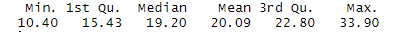This summary lists down features like Mean, Median, Minimum Value, Maximum Value and Quadrant values of the particular column.

#### Box Plotting

A box plot generate a rectangle that covers the area spanned by the column of the dataset. It can be produced as follows:

```> boxplot(mtcars\$mpg, col="green")
```

Output: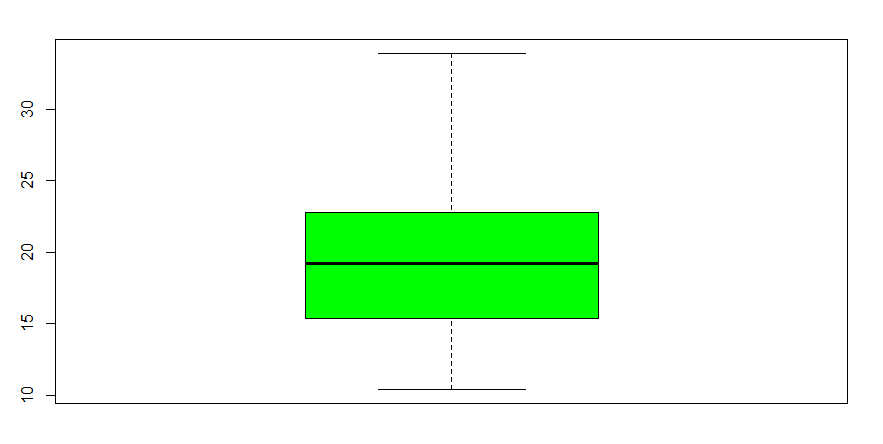Note that the thick line in the rectangle depicts the median of the mpg column, i.e. 19.20 as seen in the Five Point Summary. The col=”green” simply colors the plot green.

#### Histograms

Histograms are the most widely used plots for analyzing datasets. Here is how we can plot a histogram that maps a variable (column name) to its frequency-

```> hist(mtcars\$mpg, col = "green")               ## Plot 1
> hist(mtcars\$mpg, col = "green", breaks = 25)  ## Plot 2
> hist(mtcars\$mpg, col = "green", breaks = 50)  ## Plot 3
```

The ‘breaks’ argument essentially alters the width of the histogram bars. It is seen that as we increase the breaks value, the bars grow thinner.

Outputs: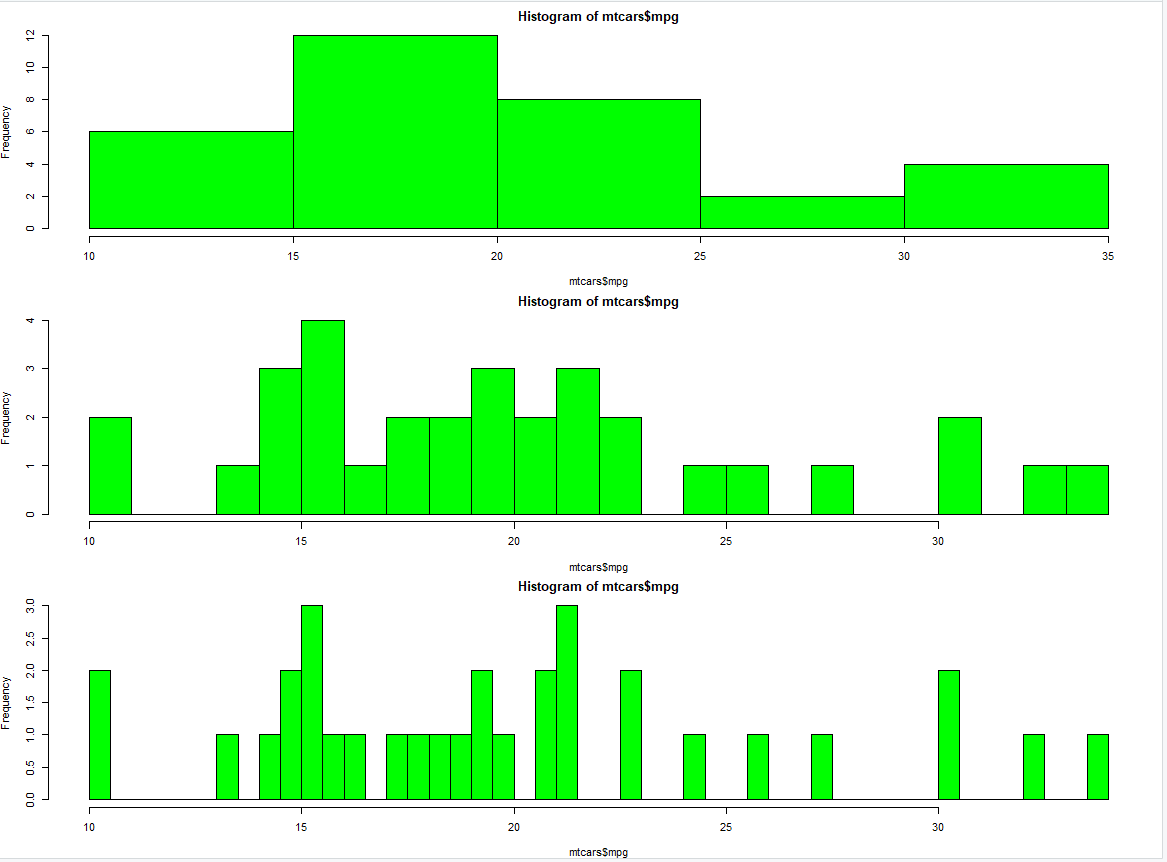#### Bar Plotting

In bar graphs, we get a discrete value-frequency mapping for each value present in the variable (column). For example –

```> barplot(table(mtcars\$carb), col="green")
```

Output: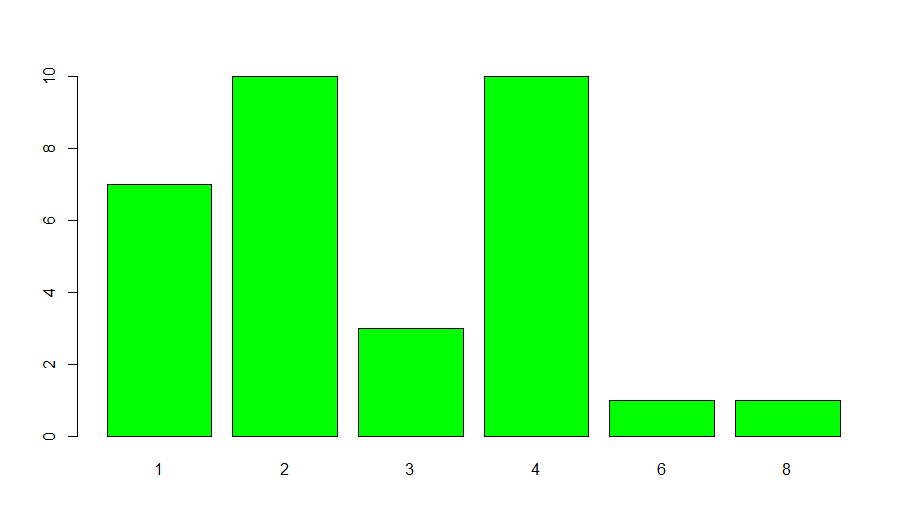We see that the column ‘carb’ contains 6 discrete values (in all its rows). The above bar graph maps these 6 values to their frequency (the number of times they occur).

### Two-Dimensional Plotting

In two-dimensional plotting, we visualize and compare one variable with respect to the other.

#### Box Plotting

Suppose we wish to generate multiple boxplots, on the basis of the number of gears that each car has. So, the number of boxplots we wish to have is equal to the number of discrete values in the column ‘gear’, i.e. one plot for each value of the gear. This can be achieved in the following way –

```> boxplot(mpg~gear, data=mtcars, col = "green")
```

Output: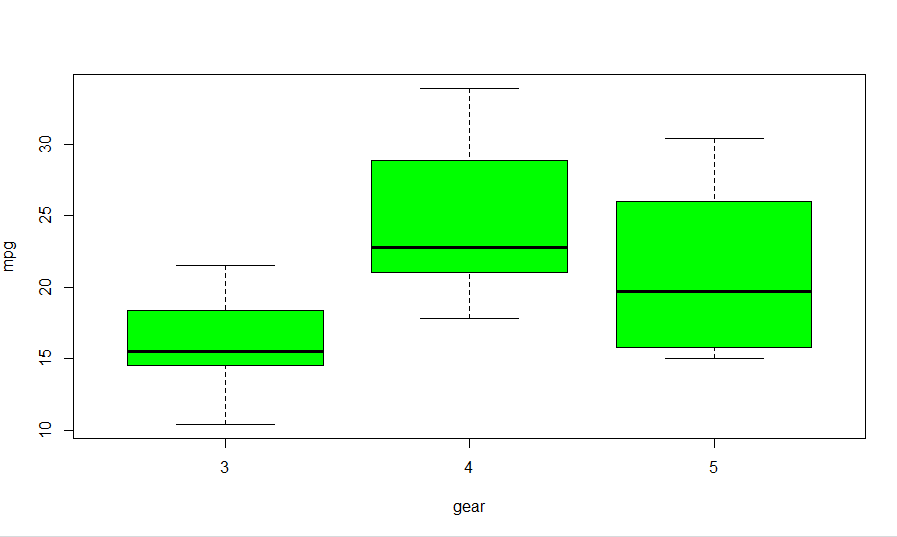We see that there are 3 values of gears in the ‘gear’ column. So, 3 different box-plots, one for each gear have been plotted.

#### Histograms

Now suppose, we wish to create separate histograms for cars that have 4 cylinders and cars that have 8 cylinders. To do this, we subset our dataset such that the subset data contains data only for those cars which have 4 (or 8) cylinders. Then, we can easily plot our subset data using hist() function as before. This is how we can achieve this –

```> hist(subset(mtcars, cyl == 4)\$mpg, col = "green")        ## Plot 1
> hist(subset(mtcars, cyl == 8)\$mpg, col = "green")        ## Plot 2
```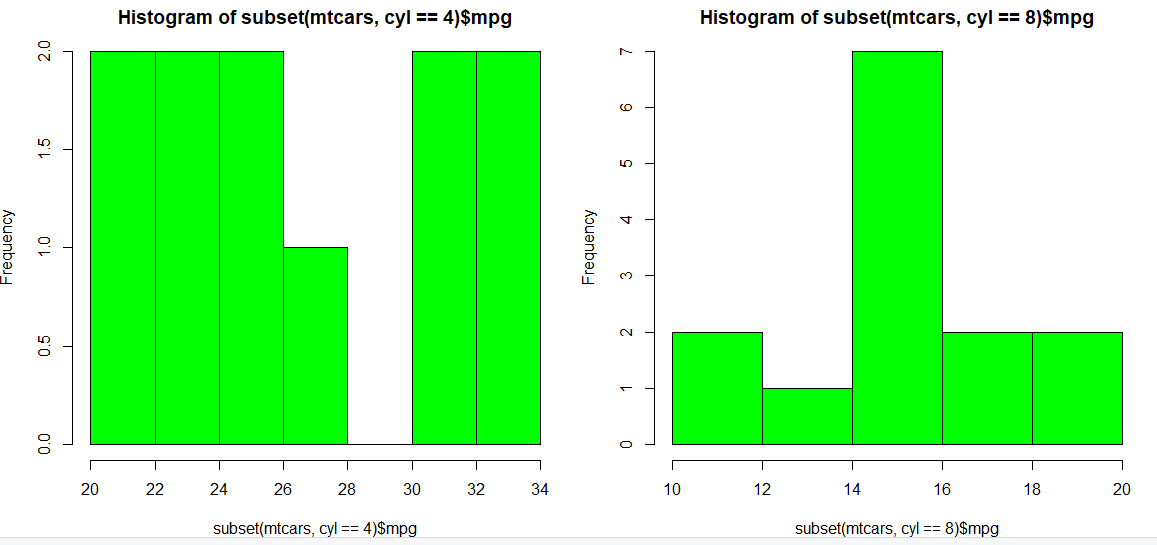#### Scatter Plot

Scatter plots are used to plot data points for two variables on the x and y-axis. They tell us patterns amongst data and are widely used for modeling ML algorithms. Here, we scatter plot the column qsec with respect to the column mpg.

```> with(mtcars, plot(mpg, qsec))
```

Output: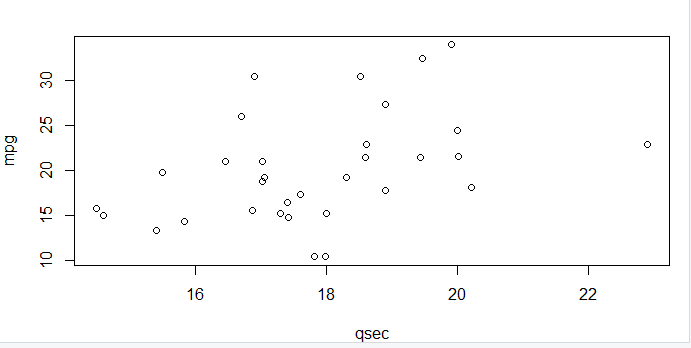However, the above plot does not really show us any patterns in data. This is because of the limited number of rows (samples) we had in our dataset. When we obtain data from external resources, it normally has a minimum of 1000+ rows. On plotting such an extensive dataset on a scatter plot, we pave way for really interesting observations and insights.

My Personal Notes arrow_drop_up
Recommended Articles
Page :